### The Bohr Atom *

Bohr postulated that electrons orbited the nucleus like planets orbiting the sun. He managed to fit the data for Hydrogen by postulating that electrons orbited the nucleus in circular orbits, and that angular momentum is quantized such that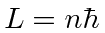, for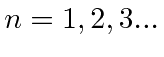. This is natural since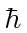has units of angular momentum. Bohr correctly postdicted the Hydrogen energies and the size of the atom.

Balance of forces for circular orbits.Angular momentum quantization assumption.Solve for velocity.Plug into force equation to get formula for.Now we just want to plugandinto the energy formula. We write the Hydrogen potential in terms of the fine structure constant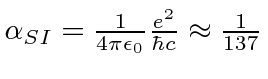.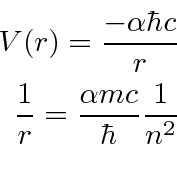We now compute the energy levels.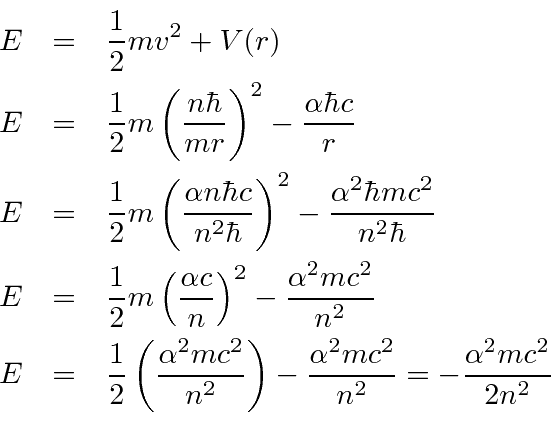The constanteV. Bohr's formula gives the right Hydrogen energy spectrum.

We can also compute the ground state radius of the Bohr orbit.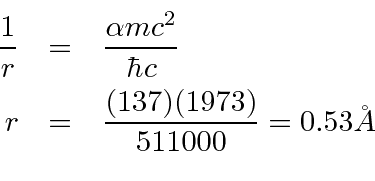This is also about the right radius. The name for this number, the Bohr radius, persists to this day despite the fact that Bohr's assumption is wrong.

Although angular momentum is quantized in units of, the ground state of Hydrogen has zero angular momentum. This would put Bohr's electron in the nucleus.

Bohr fit the data, with some element of truth, but his model is WRONG.

Jim Branson 2013-04-22